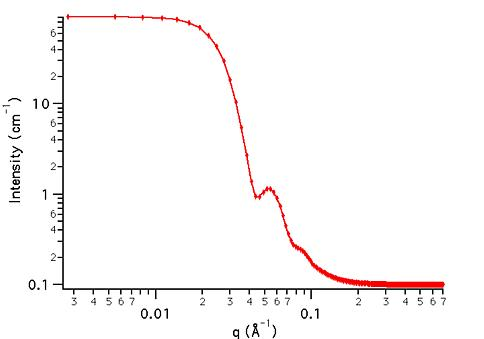MODEL

PolyHardSphere

AUTHOR/MODIFICATION

Steve Kline 06 NOV 1998

Alan Munter 08 JULY 1999, converted to Java.

APPROVED FOR DISTRIBUTION

DESCRIPTION

This function calculates the scattered intensity for a population of polydisperse spheres, including hard sphere interactions between the particles. The calculation is an exact, multicomponent solution, using the Percus-Yevick closure. A Schulz distribution is used to describe the polydispersity of the diameter.

VARIABLES

Input Variables (default values):

Parameter Variable Value
1Polydispersity (0-1)0.12
2Volume Fraction (0-1)0.1
3Contrast (Å-2)2.0e-6
4incoherent Background(cm-1)0.000

USAGE NOTES

The returned value is the scattered intensity in absolute scale, units of [cm-1]. Since both the form factor and structure factor are included in this calculation, any other structure factor selected in the calculation will not be applied.

Polydispersity, p = s/R, where s2 is the variance of the distribution and R is the mean particle radius, Parameter. For a more complete description of the Schulz distribution, see: J. Hayter in "Physics of Amphiphiles - Micelles, Vesicles and Microemulsions" V. DeGiorgio and M. Corti, Eds. (1983) p. 69.

Polydispersity, Parameter, is constrained during calculation to remain between its physical limits of 0 < p < 1. If a value larger than this is entered the value is quietly converted to 1.

Scattering contrast = SLD (sphere) - SLD (solvent).

Volume fraction and scattering contrast are correlated, and one or both should be held fixed during model fitting.

REFERENCE

Griffith, W. L.; Triolo, R.; Compere, A. L. Phys. Rev. A, 1987, 35, 2200.

TEST DATASET

This example dataset is produced by calculating the PolyHardSphere using 256 data points, qmin = 0.001 Å-1, qmax = 0.7 Å-1 and the above default parameter values.